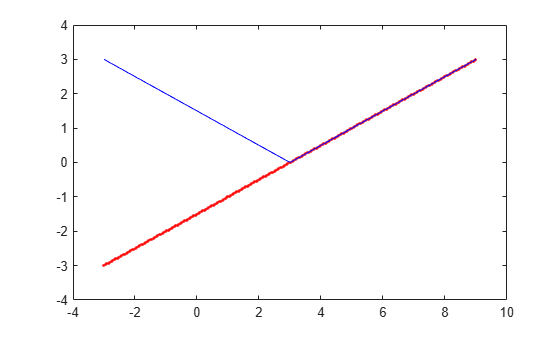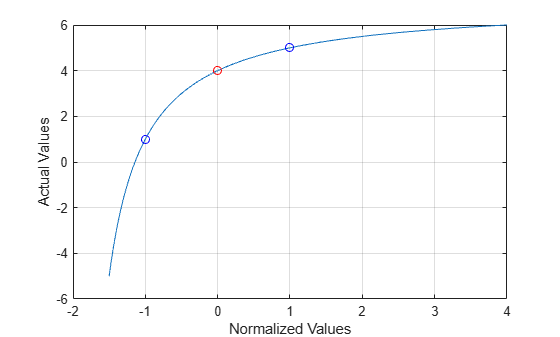actual2normalized

Transform actual values to normalized values

Syntax

NV = actual2normalized(uElement,AV)
[NV,ndist] = actual2normalized(uElement,AV)

Description

NV = actual2normalized(uElement,AV) transforms the values AV of the uncertain element uElement into normalized values NV. If AV is the nominal value of uElement, NV is 0. Otherwise, AV values inside the uncertainty range of uElement map to the unit ball ||NV|| <= 1, and values outside the uncertainty range map to ||NV|| > 1. The argument AV can contain a single value or an array of values. NV has the same dimensions as AV.

[NV,ndist] = actual2normalized(uElement,AV) also returns the normalized distance ndist between the values AV and the nominal value of uElement. This distance is the norm of NV. Therefore, ndist <= 1 for values inside the uncertainty range of uElement, and ndist > 1 for values outside the range. If AV is an array of values, then ndist is an array of normalized distances.

The robustness margins computed byrobstab and robgain serve as bounds for the normalized distances in ndist. For example, if an uncertain system has a stability margin of 1.4, this system is stable for all uncertain element values whose normalized distance from the nominal is less than 1.4.

Examples

collapse all

For uncertain real parameters whose range is symmetric about their nominal value, the normalized distance is intuitive, scaling linearly with the numerical difference from the uncertain real parameter's nominal value.

Create uncertain real parameters with a range that is symmetric about the nominal value, where each end point is 1 unit from the nominal. Points that lie inside the range are less than 1 unit from the nominal, while points that lie outside the range are greater than 1 unit from the nominal.

a = ureal('a',3,'range',[1 5]);
NV = actual2normalized(a,[1 3 5])
NV = 1×3

-1.0000         0    1.0000

NV = actual2normalized(a,[2 4])
NV = 1×2

-0.5000    0.5000

NV = actual2normalized(a,[0 6])
NV = 1×2

-1.5000    1.5000

Plot the normalized values and normalized distance for several values.

values = linspace(-3,9,250);
[nv,ndist] = actual2normalized(a,values);
plot(values,nv,'r.',values,ndist,'b-')Create a nonsymmetric parameter. The end points are 1 normalized unit from nominal, and the nominal is 0 normalized units from nominal. Moreover, points inside the range are less than 1 unit from nominal, and points outside the range are greater than 1 unit from nominal. However, the relationship between the normalized distance and numerical difference is nonlinear.

au = ureal('ua',4,'range',[1 5]);
NV = actual2normalized(au,[1 4 5])
NV = 1×3

-1     0     1

NV = actual2normalized(au,[2 4.5])
NV = 1×2

-0.8000    0.4000

NV = actual2normalized(au,[0 6])
NV = 1×2

-1.1429    4.0000

Graph the relationship between actual and normalized values. The relationship is very nonlinear.

AV = linspace(-5,6,250);
NV = actual2normalized(au,AV);

plot(NV,AV,0,au.NominalValue,'ro',-1,au.Range(1),'bo',1,au.Range(2),'bo')
grid, xlabel('Normalized Values'), ylabel('Actual Values')The red circle shows the nominal value (normalized value = 0). The blue circles show the values at the edges of the uncertainty range (normalized values = -1, 1).

Algorithms

For details on the normalize distance, see Normalizing Functions for Uncertain Elements.